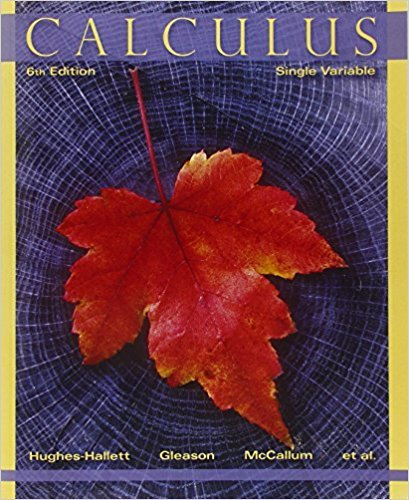×
×

# Solutions for Chapter 3.3: THE PRODUCT AND QUOTIENT RULES## Full solutions for Calculus: Single Variable | 6th Edition

ISBN: 9780470888643Solutions for Chapter 3.3: THE PRODUCT AND QUOTIENT RULES

Solutions for Chapter 3.3
4 5 0 395 Reviews
10
2
##### ISBN: 9780470888643

This expansive textbook survival guide covers the following chapters and their solutions. This textbook survival guide was created for the textbook: Calculus: Single Variable , edition: 6. Calculus: Single Variable was written by and is associated to the ISBN: 9780470888643. Since 74 problems in chapter 3.3: THE PRODUCT AND QUOTIENT RULES have been answered, more than 32451 students have viewed full step-by-step solutions from this chapter. Chapter 3.3: THE PRODUCT AND QUOTIENT RULES includes 74 full step-by-step solutions.

Key Calculus Terms and definitions covered in this textbook

If u = v and w = z , then u + w = v + z

• Arccosecant function

See Inverse cosecant function.

• Blocking

A feature of some experimental designs that controls for potential differences between subject groups by applying treatments randomly within homogeneous blocks of subjects

• Boundary

The set of points on the “edge” of a region

• Bounded

A function is bounded if there are numbers b and B such that b ? ƒ(x) ? B for all x in the domain of f.

• Categorical variable

In statistics, a nonnumerical variable such as gender or hair color. Numerical variables like zip codes, in which the numbers have no quantitative significance, are also considered to be categorical.

• Damping factor

The factor Ae-a in an equation such as y = Ae-at cos bt

• equation of a parabola

(x - h)2 = 4p(y - k) or (y - k)2 = 4p(x - h)

• Frequency table (in statistics)

A table showing frequencies.

• Graph of parametric equations

The set of all points in the coordinate plane corresponding to the ordered pairs determined by the parametric equations.

• Index

• Inductive step

See Mathematical induction.

• NDER ƒ(a)

See Numerical derivative of ƒ at x = a.

• Partial fraction decomposition

See Partial fractions.

• Pie chart

See Circle graph.

• Polar axis

See Polar coordinate system.

• Projection of u onto v

The vector projv u = au # vƒvƒb2v

• Solve a system

To find all solutions of a system.

• Solve by substitution

Method for solving systems of linear equations.

• Ymin

The y-value of the bottom of the viewing window.

×